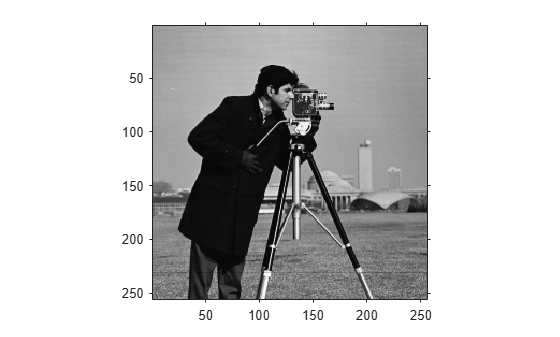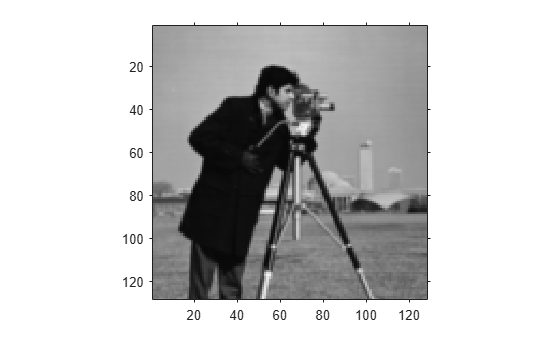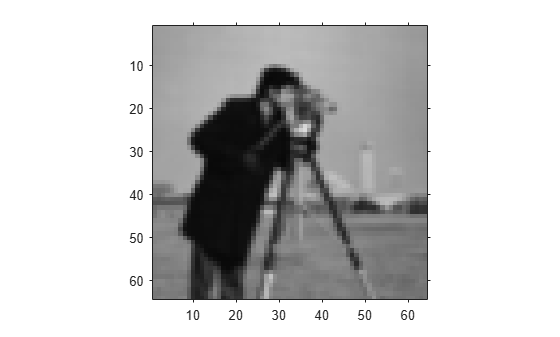impyramid

Image pyramid reduction and expansion

Description

example

B = impyramid(A,direction) computes a Gaussian pyramid reduction or expansion of A by one level. direction determines whether impyramid performs a reduction or an expansion.

Examples

collapse all

Perform a series of reductions. The first call reduces the original image. The other calls to impyramid use the previously reduced image.

I1 = impyramid(I, 'reduce');
I2 = impyramid(I1, 'reduce');
I3 = impyramid(I2, 'reduce');

View the original image and the reduced versions.

figure, imshow(I)figure, imshow(I1)figure, imshow(I2)figure, imshow(I3)Input Arguments

collapse all

Image to reduced or expanded, specified as a numeric or logical array.

Data Types: single | double | int8 | int16 | int32 | uint8 | uint16 | uint32 | logical

Reduction or expansion, specified as one of the following values:

ValueDescription
'reduce'Return an image, smaller than the original image.
'expand'Return an image that is larger than the original image.

Data Types: char | string

Output Arguments

collapse all

Reduced or expanded image, returned as a numeric or logical array, the same class as A.

Algorithms

If A is m-by-n and direction is 'reduce', the size of B is ceil(M/2)-by-ceil(N/2). If direction is 'expand', the size of B is (2*M-1)-by-(2*N-1).

Reduction and expansion take place only in the first two dimensions. For example, if A is 100-by-100-by-3 and direction is 'reduce', then B is 50-by-50-by-3.

impyramid uses the kernel specified on page 533 of the Burt and Adelson paper:

$w=\left[\frac{1}{4}-\frac{a}{2},\frac{1}{4},a,\frac{1}{4},\frac{1}{4}-\frac{a}{2}\right]$, where . The parameter a is set to 0.375 so that the equivalent weighting function is close to a Gaussian shape. In addition, the weights can be readily applied using fixed-point arithmetic.

 Burt and Adelson, "The Laplacian Pyramid as a Compact Image Code," IEEE Transactions on Communications, Vol. COM-31, no. 4, April 1983, pp. 532-540.

 Burt, "Fast Filter Transforms for Image Processing," Computer Graphics and Image Processing, Vol. 16, 1981, pp. 20-51